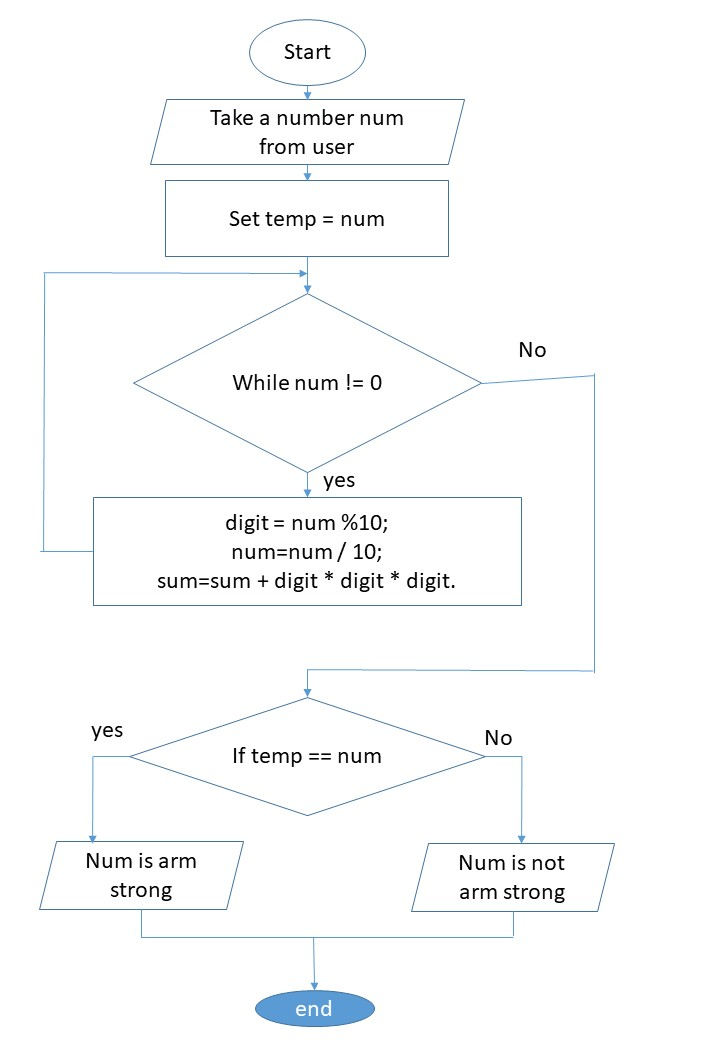# Write a program to find whether the number is Armstrong number

• Introduction
• Algorithm of Problem
• Flow Chart
• Program in C
• Out Put

## Introduction

Armstrong number is the number whose digits cube is sum together we get the same number ex 370 in this number we have three digits 3 , 7 , 0, if we calculate the cube of each digit cube of 3 is 27, similarly cube of 7 is 343 and 0 is 0. if we add the resulted value 343+27+0=370 as answer is same as number so number is Armstrong number.

## Algorithm of solving given problem

Step 1: Declare three variable digit, sum , num
Step 2: Take  a number as input from user
Step 3: initialize the value of sum = 0 and set temp = num
Step 4: Repeat step 5 to 7  until num !=0
Step 5: Set digit = num %10;
Step 6: Set num=num / 10;
Step 7: Set sum=sum + digit * digit * digit.
Step 8: If sum == temp than
print number is arm strong
otherwise
print number is not arm strong
Step 9: Exit

## Flow ChartFlow chart

## Program in C language

#include<stdio.h>
#include<conio.h>

void main()
{
int digit,sum=0,temp,num;
printf("enter an number\n");
scanf("%d",&num);
temp=num;
while(num!=0)
{
digit=num%10;
num=num/10;
sum=sum+digit*digit*digit;
}
if(sum==temp)
{
printf("number is Armstrong");
}
else
{
printf("number is not Armstrong");
}

}

OUTPUT

enter an number
370
number is Armstrong

enter an number
370
number is not Armstrong